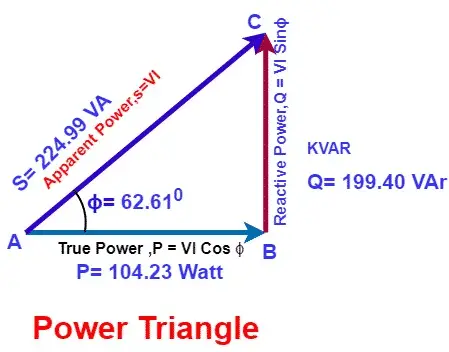# What is a Power Triangle? Active, Reactive & Apparent Power

Power triangle represents the active power, reactive power and apparent power of AC circuit in aright angle triangle. The three sides of the right angle triangle shows the relationship between all three powers. The power triangle is a useful tool for calculating the power- active, reactive and apparent power in a AC circuit if two out of three power is known. There are many combinations of electrical  load like pure resistive, inductive capacitive or combination of RL,RC,RLC,LC etc. The inductive, and capacitive load draws a reactive power from the source and feed back the power to source again.

Lagging or leading of the circuit current depends on the types of electrical load. The pure resistive circuit draw the current which is in phase with the applied voltage. The voltage and current are in phase in the resistive circuit.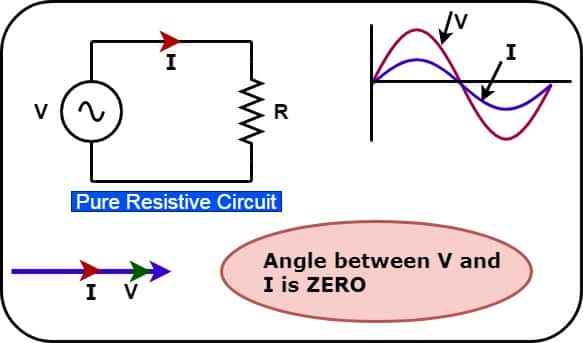The pure inductive circuit draw the current which lags the applied voltage 90 electrical degree.The phasor diagram of voltage and current for pure inductive load and RL load is given below.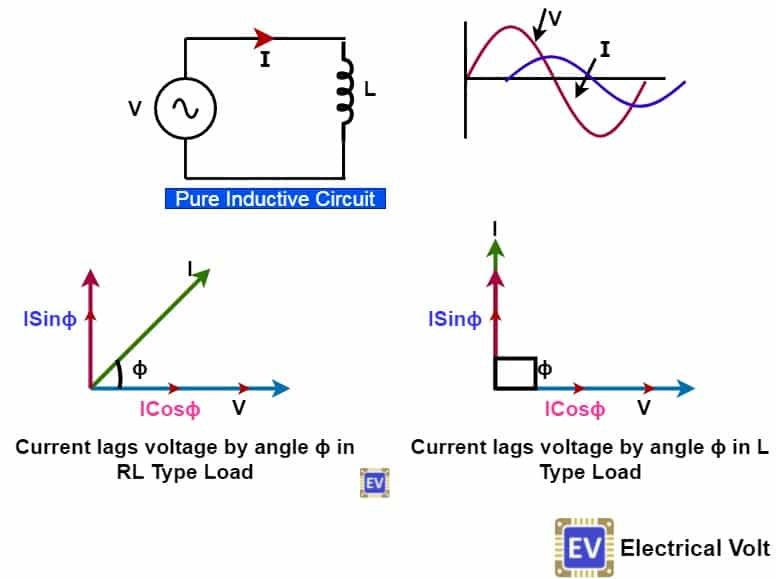And, the pure capacitive  circuit draw the current which lead the applied voltage 90 electrical degree. The leading or lagging current cause reactive current in the circuit.The phasor diagram of voltage and current for pure capacitive load and RC load is given below.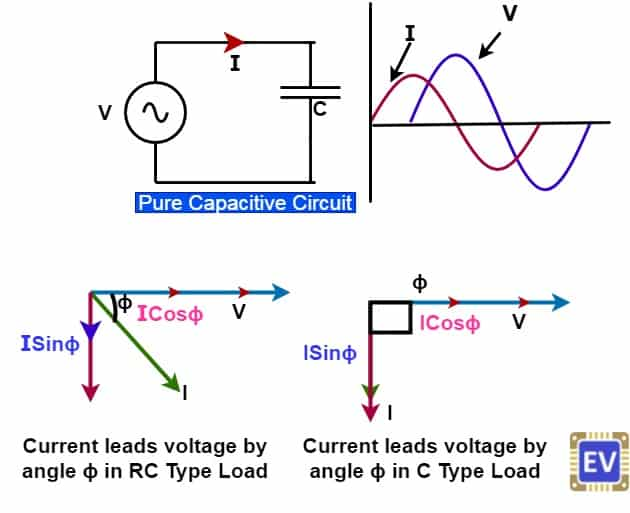From above phasor diagram, it is clear that inductive and capacitive circuit draw reactive current. The total current in the circuit is thus the vector sum of active current and reactive current. We can show the active,reactive and apparent power by a right angle triangle.

The reactive component draws a reactive current and its magnitude is ISinɸ. The active component draws active current, and its magnitude is  I Cosɸ. The Product of the reactive component of current ( ISinɸ) and voltage(V) is the reactive power. The Product of the active component of current  ( I Cosɸ), and voltage(V) is the active power. Resultant vector sum of the active and reactive power is the apparent power.

### What is a Power Triangle?

We represent these three powers of AC circuit by a triangle in a following ways.
Triangle  Base                   = Active Power
Perpendicular  = Reactive Power
Hypotenuse     = Apparent Power
Now, we can represent all three powers active power, reactive power and apparent power on the sides of a right angle triangle.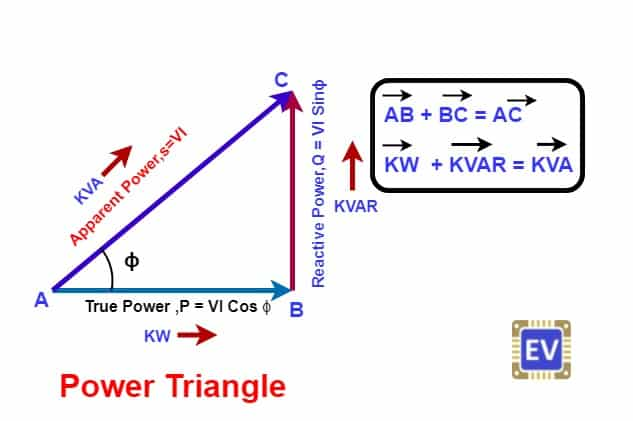The actual power consumed by the load is active power or real power. The active power is the energy consumed by the load. The active power is measured in Watt, KW or MW.
 Active Power (P) = VI Cosɸ                 [ Cosɸ = Power Factor ]
The reactive elements are capacitor and inductor. These circuit elements draw reactive power from the supply source. The reactive current lags or leads with the applied voltage. These circuit elements draws current in positive half cycle and the delivers the same to the supply source in the negative half cycle. Thus, the reactive power back and forth in the reactive circuit. Therefore, the reactive power is watt less power and, it does not do any useful work. It is measured in VAR, Kilowatt-ampere (KVAR) or MVAR.
 Reactive Power (Q)  = VI Sinɸ
The reactive power is the watt less, however it draws the current from the supply source, and eventually it increase the total circuit current. Therefore, the total current is very important for designing of the electrical circuit. If we vectorially add the active power and reactive power, the sum is equal to the apparent power(S).Apparent power is measured in VA, KVA or MVA.
 Apparent Power(S ) = VI*
I* is the vector sum of active and reactive current.
We can calculate the apparent power by a power triangle if active and reactive power data is available. The formula of apparent power according to power triangle is ;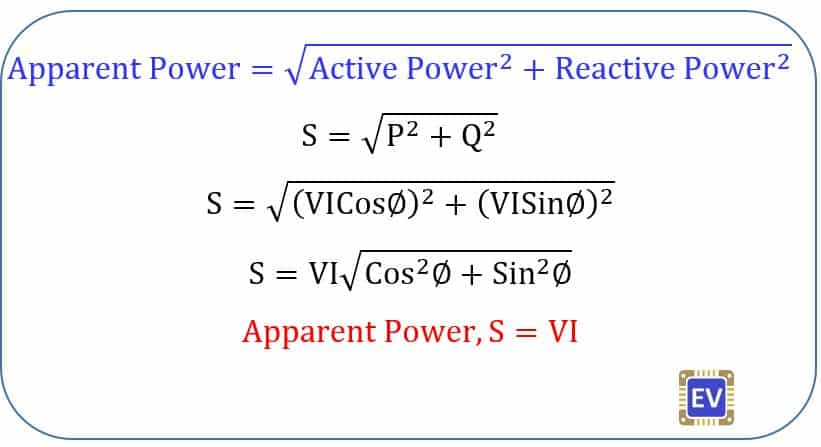We can find the power of the AC circuit by power triangle, if two out of three powers is available. Then , all three powers can be drawn on power triangle to show the relationship between the active power, reactive power and apparent power.

Summary

1.  We denote the active power on the base of power triangle. The watt meter measures the  active  power. The active power is the useful power used by the equipment to do useful work. The examples of the equipment drawing are electrical motor, oven, heater,geyser etc.
2. The reactive power (inductive type) is used for electrical machines’ functioning.  The induction motor draws inductive reactive power to produce rotating magnetic field. Similarly, transformer also draw reactive power to set up magnetic flux in its core. The reactive power is necessary evils. Without consuming reactive power, an electrical machine can not work. The cause of poor power factor is drawing of more reactive power from the supply source.
3. To improve the power factor of the system, we add capacitor banks to nullify the effect of inductive reactive power. The capacitor banks draws reactive power in just phase opposition of the inductive reactive power, and thus the net reactive power of the circuit decrease.
4. The apparent power shows the total circuit current whether its is active or reactive. There is endeavors to reduce the apparent power for economic operation of the electrical network. The less the reactive power, the less is the losses in the transmission line.
5. After determining the active power and reactive power, the Power factor can be calculated using the power triangle.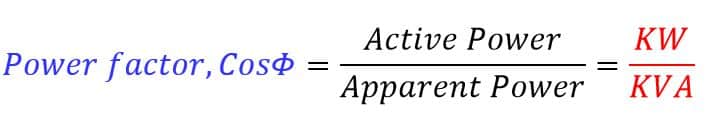### Power Triangle Example No. 1

A wound coil that has an inductance of 150mH and a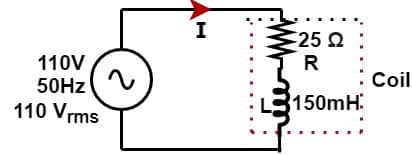resistance of 25 Ω is connected to a 110V 50Hz supply.
Calculate:
a) the impedance of the coil, b) the current,c) the power factor,and d) the apparent power consumed.
Also draw the resulting power triangle for the above coil.

a) The impedance of the coil
R = 25 Ω
XL = 2πfL = 2 x 3.14 x 50 x 150 x 10-3 = 47.1Ω
Z = √ ( R2 + XL2)   = √ ( 252 + 47.12)  = 53.32 Ω
The impedance of the coil is  Z = 53.32 Ω

b) The Current through the Coil
I = V/Z = 110/53.32 = 2.06 A
c) Power Factor
Cosɸ = R/Z = 25/53.32 = 0.46
ɸ  = Cos-1 (0.46) = 62.61°
d) Apparent Power
Cosɸ = 0.46
Sinɸ = √ ( 1 – Cos2ɸ) = √ ( 1 – 0.462) = 0.88
Active Power = VI Cosɸ = 110 x 2.06 x 0.46 = 104.23 Watt
Reactive Power = VI Sinɸ = 110 x 2.06 x 0.88 = 199.40  VAr
Apparent Power = √ ( Active Power2 + Reactive Power2 )
= √ ( 104.232 + 199.402 ) =224.99 VA
Apparent Power = 224.99 VA
Power Triangle for the coil